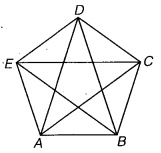# Class 6 Maths NCERT Solutions for Chapter 5 Understanding Elementary Shapes Ex – 5.8

## Understanding Elementary Shapes

Question 1.
Examine whether the following are polygons. If any one among them is not, say why?Solution:
(a) No, because it is not closed,
(b) Yes.
(c) No, because it is not drawn by using line segments.
(d) No, because it is not closed by line segments.

Question 2.
Name each polygon: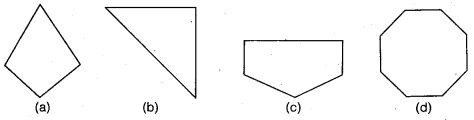make two more examples of each of these.

Solution:
(b) Triangle
(c) Pentagon
(d) Octagon

Two more examples of :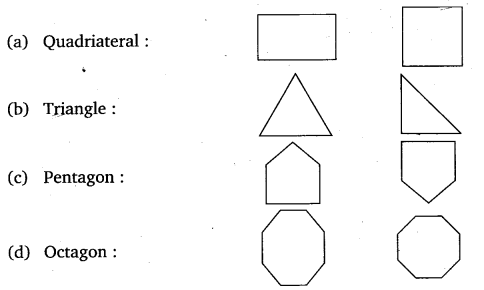Question 3.
Draw a rough sketch of a regular hexagon. Connecting any three of its vertices, draw a triangle. Identify the type of the triangle you have drawn.

Solution: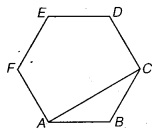Let ABCDEF be a rough sketch of a regular hexagon.
Join AC
A ∆ ABC is obtained in which AB = BC. Thus,
∆ ABC is an isosceles triangle.

Question 4.
Draw a rough sketch of a regular octagon.

(Use squared paper if you wish). Draw a rectangle by joining exactly four of the vertices of the octagon.

Solution:
Let ABCDEFGH be a rough sketch of a regular octagon. Join AC, CE, EG and GA to obtain a rectangle ACEG.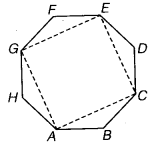Ex 5.8 Class 6 Maths Question 5.
A diagonal is a line segment that joins any two vertices of the polygon and is not a side of the polygon. Draw a rough sketch of a pentagon and draw its diagonals.
Solution:
Let ABCDE be a rough sketch of a pentagon. To draw its diagonals, join AC, AD, BD, DE and CE. Then, AC, AD, BD, BE and CE are its diagonals.